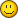# [請教] 量電阻問題?

 想用8051去量電阻... 但係上網搵左好多料都未有頭水! 淨係知應該用ADC去搵volt... 但係個慨念唔太清楚, 唔知有冇可以俾到d料我?? 唔該!!買本8051 書 就有說 有電路圖又程式仲有說明 好過上來問
 本帖最後由 oYueno 於 2010-8-6 13:33 編輯2010-8-6 13:32 設電阻測試範圍為0~5K: 1: 選47K+RV1=50K ,則I=5V/50K=0.1mA,當待測電阻Rx 最大為5k時. 則V1=0.1mA X 5K =0.5V ,V OUT=4.9V ,電壓增益Av=4.9V/0.5V=9.8 2: Av=(+1 (2R2/RV2)) X(R4/R3)=9.8 如果R2=R3=R4=10K 則20/VR2=8.8 VR2=2.27K